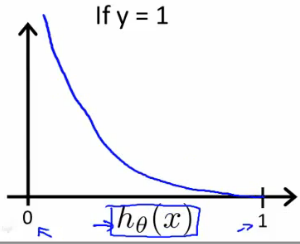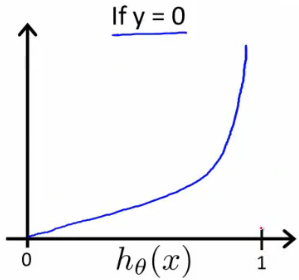The contents are about notes and equations taken from 《Machine Learning》, which is taught by Andrew Ng, in Coursera.

key words: classification

### Classification and Representation

#### Logistic Function

Our new form uses the Sigmoid Function, also called the Logistic Function.

#### Cost Function

Compared with the two equations above, we have another equivalent function:

When $y = 1$, we get the following plot for $J(\theta) \; vs \; h_\theta(x)$:Logistic regression cost function positive class

Similarly, when $y\;=\; 0$, we get the following plot for $J(\theta) \; vs \; h_\theta(x)$:Logistic regression cost function negative class

### Multiclass Classification

------本文结束感谢您的阅读 ------

0%